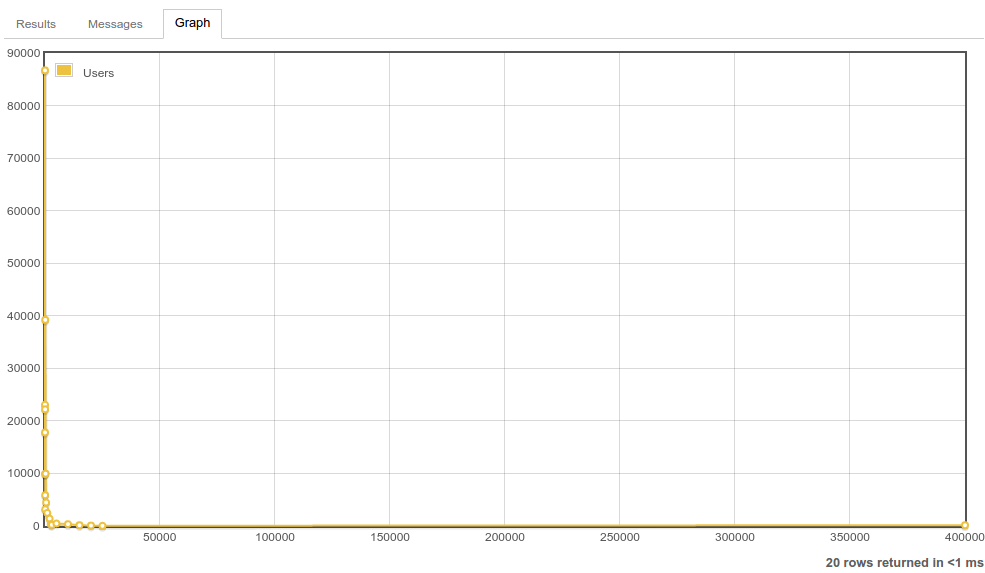<![CDATA[Category: Octave | Blog 1]]> 2017-01-28T23:37:00+08:00 https://vincenttam.github.io/ Octopress <![CDATA[Generate $\rm \LaTeX$ Code for Octave Matrices]]> 2016-01-16T15:33:39+08:00 https://vincenttam.github.io/blog/2016/01/16/generate-latex-code-for-octave-matrices Background

## Problem

Recently, I encountered a linear algebra problem on Mathematics Stack Exchange.

For any given matrix $A$ in Octave,

A = [1 2 2; 2 3 4; 4 4 2]
A =

1   2   2
2   3   4
4   4   2


how can one generate its $\rm \LaTeX$ code

so that one gets

$A = \begin{bmatrix} 1&2&2\\ 2&3&4\\ 4&4&2 \end{bmatrix}?$

## Solution

I searched “octave to latex matrices” and I found this answer quite useful. I issued the first command to see the result.

strrep(strrep(mat2str(A),",","&"),";","\\\\\n")(2:end-1)
ans = 1 2 2\\
2 3 4\\
4 4 2


I suspected that it didn’t work. I posted it as a comment and verified that I was right. To fix this, I extracted the function mat2str(A) in the middle of this command to see the results. From its result (ans = [1 2 2;2 3 4;4 4 2]), I realised that strrep standed for “string replace”. Then, I changed the double-quoted comma in the second argument of function strrep to a double-quoted whitespace character, and got the anticipated result.

strcat("\\begin{bmatrix}\n",strrep(strrep(mat2str(A)," ","&"), ...
";","\\\\\n")(2:end-1),"\n\\end{bmatrix}\n")
ans = \begin{bmatrix}
1&2&2\\
2&3&4\\
4&4&2
\end{bmatrix}


I don’t know how to do this for matrices with fractions. I think I can work it out by searching and testing in several hours, but I don’t have the time to do so.

## Lessons learnt

### Math

I revised some definitions in linear algebra.

• A matrix $U$ is unitary iff $UU^\star = U^\star U = I$.
• A matrix $N$ is normal iff $NN^\star = N^\star N$.

### GNU Octave

• The function strrep(str,"foo","bar") replaces all instances of foo in str with bar. It can be used in a nested manner for multiple replacements.
• The function strcat(str1,str2,...) concatenates the strings inside.

### Grep

When I was writing this article, I wanted to search for “octave” (with the square brackets “[]”) with grep inside Vim, but I got over 1270 results. I tried adding single/double quotes and escaping the square brackets with a backslash, but I faied again. Finally, I googled “grep escape character” for a solution. Since then, I know that I should add the -F flag to grep to fix the string.

]]>
<![CDATA[Generate Simplex Tableaux With Octave]]> 2016-01-16T14:53:15+08:00 https://vincenttam.github.io/blog/2016/01/16/generate-simplex-tableaux-with-octave Background

It’s easy that one makes careless mistakes in a pivot operation. As a result, in test/exams in which calculators are allowed, I used a simple program to save time.

Answering linear programming question on Mathematics Stack Exchange, I used GNU Octave to do the tedious work.

## Initial tableau

First write the LPP in standard form. I assume that $b$ and $c$ are column vectors.

• c is the objective function.
• A is the coefficient matrix of the constraints. (a.k.a technology matrix)
• b is the RHS of the constraints.
• T0 is the initial tableau.

My habit is to

1. place $b$ on the RHS;
2. place the objective function row at the bottom;
3. omit the coefficient for $z$ since it’ll never be changed.

## Current simplex tableau

The command basis = [3 2 6] is used to choose the decision variables $x_3,x_2$ and $x_6$ as the basis. Note that the order of the entries in the array basis is very important. By setting this array, I don’t need to repeat typing the same set of numbers for $B$ and $c_B$.

Since I’m no longer in an LP course, I’m too lazy to write the code for finding the suitable elements for a pivot operation. We don’t need to re-develop something that has been well-developed.

]]>
<![CDATA[Distribution of User Reputation on Math Stack Exchange]]> 2016-01-07T17:34:38+08:00 https://vincenttam.github.io/blog/2016/01/07/distribution-of-user-reputation-on-math-stack-exchange Background

Recently, I post math on Mathematics Stack Exchange instead of here.

## Problem

How can one get a table for the distribution of reputation on that site?

## Solution

Write a SQL query on Stack Exchange Data Explorer.

The indentation is automatically done by Vim. I know that the syntax is ugly. If I assign text string to the column u.range, then the table is sorted in alphabetical order of that column instead of numerical order. This doesn’t make sense. Therefore, I use a dirty way to get the statistics, and played with the built-in graphing function. However, the visual result isn’t so satisfactory.Anyone who has completed high school will realise that a log graph is better. Asking for this feature on Meta Stack Exchange takes time. I believe that such feature request will be rejected by the moderator to reduce the workload of Stack Exchange company. Therefore, I plot the log graph using GNU Octave.

2. Change it to an GNU Octave script file.
3. Open it using Vim.
4. Do the necessary text substitutions so that the data becomes a matrix.
5. Complete the script file by adding the plot commands.

I choose loglog because semilogx causes the labels on tail to overlap. Here’s the results.

</source>## Lessons learnt

I can save plots in GNU Octave as a SVG file. I know this after searching “octave export svg”. From Printing and Saving Plots, I see print -d[device], in which one can substitute the output format. For example, I used print -dsvg to generate the SVG’s shown above.

]]>
<![CDATA[Got a Warning From Octave]]> 2015-12-28T23:11:59+08:00 https://vincenttam.github.io/blog/2015/12/28/got-a-warning-from-octave Background

To verify the inequality

$\left(\sqrt{\frac{a+b}{c}}+\sqrt{\frac{b+c}{a}}+\sqrt{\frac{c+a}{b}} \right)^2\ge\frac{16}{3(a+b)(b+c)(c+a)}$

found on Math Stack Exchange, I wrote the following Octave script.

## Problem

The above script did the calculations, but a warning message appeared.

$octave -q test.m warning: function ./test.m shadows a core library function A = 0.60000 B = 0.24101 C = 31.975 ans = 24.691  How can I run this script without the warning? ## Solution Change the file name of the script to an uncommon name. $ mv test.m test1.m
\$ octave -q test1.m
A =  0.60000
B =  0.24101
C =  31.975
ans =  24.691

]]>